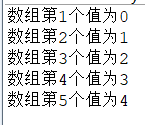# 在java中数组的输入和输出如何操作？具体步骤详解

BSO 2020-09-04 09:20:00 java常见问答 4675

java的学习是一个漫长而又持久的过程，它包含了内涵丰富意义深厚的各类知识，使得学习长久难以简化。今天就来为大家介绍一下，在java中如何实现数组的输入与输出，并且通过具体的步骤为大家展示。

1.先声明一个int型数组，如下所示：

`int[] a 或者 int a[]`

2.给数组分配空间，如下所示：

```a = new int;

3.给数组赋值，如下所示：

```a = 1; //0代表的是数组的第1个元素 ,元素下标为0
a = 1; //1代表的是数组的第2个元素 ,元素下标为0```

4.访问数组数据 ,使用数组下标访问：c=a;

```int[] a = {
12
, 3
, 4
, 8
, 5
, 6
, 6
, 7
, 8
, 8
, 9
, 8
};
int[] a1 = new int[]
{
12
, 3
, 4
, 8
, 5
, 6
, 6
, 4
};```

5.同时注意，new int[ ]的[ ]一定不能放内存大小，使用循环给数组赋值，示例如下所示：

``` int[] arr = new int;
for (int i = 0; i < arr.length; i++)
{
System.out.println("数组第" + (i + 1) + "个值为" + i);
}``````int a[] = new int;
for (int i = 1; i <= n; i++)
{
a[i] = in .nextInt();
}```

```int[] array = {
1
, 2
, 3
, 4
, 5
};```

1.传统的for循环方式，如下所示：

```for (int i = 0; i < array.length; i++)
{
System.out.println(a[i]);
}```

2.for each循环，如下所示：

```for (int a: array)
System.out.println(a);```

3.使用Array类中的toString方法，调用Array.toString(a)，然后返回一个包含数组元素的字符串，这些元素被放置在括号内，并用逗号分开。如下所示：

```int[] array = {
1
, 2
, 3
, 4
, 5
};
System.out.println(Arrays.toString(array));```

```int[][] magicSquare = {
{
16
, 3
, 2
, 13
}
, {
5
, 10
, 11
, 8
}
, {
9
, 6
, 7
, 3
}
};```

1.传统的for循环方式，如下所示：

```for (int i = 0; i < magicSquare.length; i++)
{
for (int j = 0; j < magicSquare[i].length; j++)
{
System.out.print(magicSquare[i][j] + " ");
}
System.out.println(); //换行
}```

2.for each循环，如下所示：

```for (int[] a: magicSquare)
{
for (int b: a)
{
System.out.print(b + " ");
}
System.out.println(); //换行
}```

3.利用Array类中的toString方法，如下所示：

```for (int i = 0; i < magicSquare.length; i++)
System.out.println(Arrays.toString(magicSquare[i]));```

java数组求最大值要如何实现?

java数组逆序输出代码怎么编写?

java数组升序怎么实现?